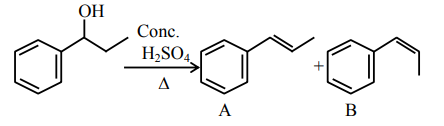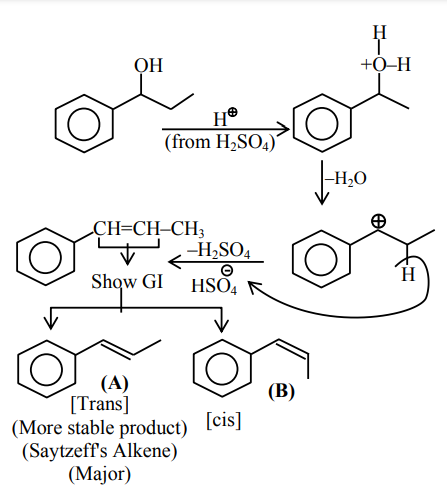# Prove the following`
Question:consider the above reaction, and choose the correct statement:

1. The reaction is not possible in acidic medium

2. Both compounds $\mathbf{A}$ and $\mathbf{B}$ are formed equally

3. Compound $\mathbf{A}$ will be the major product

4. Compound $\mathbf{B}$ will be the major product

Correct Option: 3,

Solution: